# A uniform disk turns at 2.7 rev/s around a frictionless central axis. A nonrotating rod, of the same mass as the disk a... related homework questions

• #### A uniform disk turns at 2.7 rev/s around a frictionless central axis. A nonrotating rod, of the same mass as the disk a...

A uniform disk turns at 2.7 rev/s around a frictionless central axis. A nonrotating rod, of the same mass as the disk and length equal to the disk's diameter, is dropped onto the freely spinning disk(Figure 1) . They then turn around the spindle with their centers superposed. What is the angular frequency in rev/s of the combination?

• #### A uniform disk turns at 3.6 rev/s around a frictionless central axis. A nonrotating rod, of the same mass as the dis...

A uniform disk turns at 3.6 rev/s around a frictionless central axis. A nonrotating rod, of the same mass as the disk and length equal to the disk's diameter, is dropped onto the freely spinning disk(Figure 1). They then turn around the spindle with their centers superposed. Figure 1 of 1 Part A What is the angular frequency in rev/s of the combinatio...

• #### A uniform disk turns at 3.7 rev/s around a frictionless spindle. A nonrotating rod, of the same mass as the disk and len...

A uniform disk turns at 3.7 rev/s around a frictionless spindle. A nonrotating rod, of the same mass as the disk and length equal to the disk's diameter, is dropped onto the freely spinning disk. They then turn together around the spindle with their centers superposed. What is the angular frequency in rev/s of the combination?

• #### It’s review question, I need this as soon as possible. Thank you 3) For thè diferential equation: (a) The point zo =-1 is an ordinary point. Compute the recursion formula for the coefficients of...It’s review question, I need this as soon as possible. Thank you 3) For thè diferential equation: (a) The point zo =-1 is an ordinary point. Compute the recursion formula for the coefficients of the power series solution centered at zo- -1 and use it to compute the first three nonzero terms of the power series when -1)-s and v(-1)-0. (25 points) (b) Th...

• #### A uniform disk turns at 3.6 rev/s around a frictionless spindle. A non rotating rod, of the same mass as the disk and l...

A uniform disk turns at 3.6 rev/s around a frictionless spindle. A non rotating rod, of the same mass as the disk and length equal to the disk's diameter, is dropped onto the freely spinning disk . They then both turn around the spindle with their centers superposed. What is the angular frequency in rev/s of the combination? please express answer i...

• #### Dont copié formé thé book oh ya dont copié formé thé book cause you Oiil inde up being triste soi remembré not toi copié frome thé book oh ya

Dont copié formé thé book oh ya dont copié formé thé book cause you Oiil inde up being triste soi remembré not toi copié frome thé book oh ya!translation in english please!

• #### What is the angular frequency in rev/s of the combination? A uniform disk turns at 2.4 rev/s around a frictionless sp...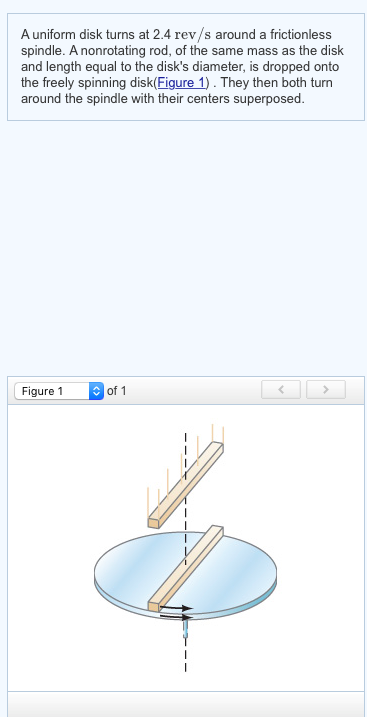What is the angular frequency in rev/s of the combination? A uniform disk turns at 2.4 rev/s around a frictionless spindle. A nonrotating rod, of the same mass as the disk and length equal to the disk's diameter, is dropped onto the freely spinning disk(Figure 1). They then both turn around the spindle with their centers superposed. Figure 1 of 1

• #### Figure 3 Uniform disk Uniform rod 3) Figure 3 illustrates a physical pendulum comprising a uniform disk having mass M and radius R and a rod having the length R and mass M. The disk is pivotally m...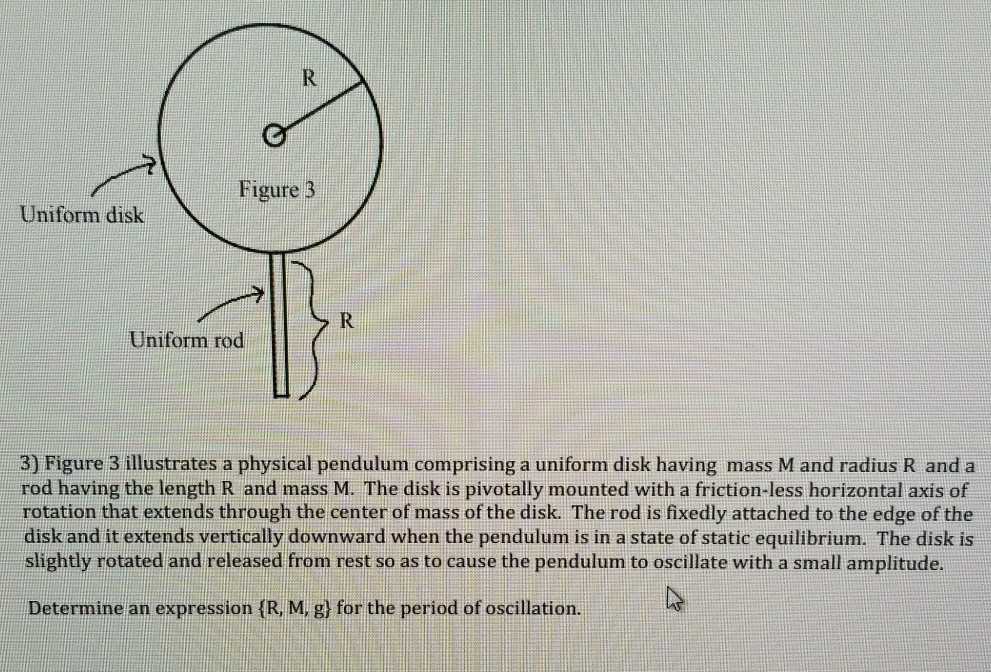Figure 3 Uniform disk Uniform rod 3) Figure 3 illustrates a physical pendulum comprising a uniform disk having mass M and radius R and a rod having the length R and mass M. The disk is pivotally mounted with a friction-less horizontal axis of rotation that extends through the center of mass of the disk. The rod is fixedly attached to the edge of the disk...

• #### A potter's wheel is rotating around a vertical axis through its center at a frequency of 2.0 rev/s . The wheel can be considered a uniform disk of mass 4.7 kg and diameter 0.30 m . The potter then throws a 2.8-kg chunk of clay, approximately shaped as a f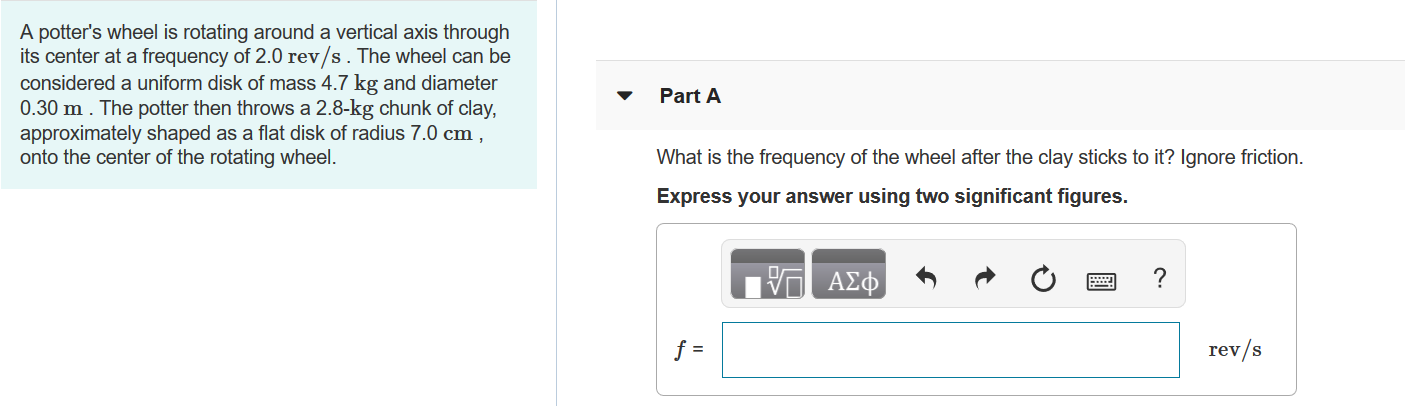A potter's wheel is rotating around a vertical axis through its center at a frequency of 2.0 rev/s . The wheel can be considered a uniform disk of mass 4.7 kg  and diameter 0.30 m . The potter then throws a 2.8-kg chunk of clay, approximately shaped as a flat disk of radius 7.0 cm , onto the center of the rotating wheel.A) What is the frequency of t...

• #### A compact disk, which has a diameter of 12.0 cm, speeds up uniformly from zero to 4.40 rev/s in 3.20 s What is the tangential acceleration of a point on the outer rim of the disk at the moment when its angular speed is 3.00 {rev/s}

A compact disk, which has a diameter of 12.0 cm, speeds up uniformly from zero to 4.40 rev/s in 3.20 s What is the tangential acceleration of a point on the outer rim of the disk at the moment when its angular speed is 3.00 {rev/s}?

• #### The Central Hydraulic Supply Company is a distributor of hydraulic supplies in Central California. Central handles standard fittings, tubing, and similar items. Generally Central carries an entire pro...

The Central Hydraulic Supply Company is a distributor of hydraulic supplies in Central California. Central handles standard fittings, tubing, and similar items. Generally Central carries an entire product line for each manufacturer it represents providing local stock for rapid delivery to customers. Central stocks parts that are used in the maintenance of la...

• #### The same as the previous question. The crank AB has a constant clockwise angular velocity of 2000 rpm. The connecting rod is a uniform slender rod whose center of mass is in its geometric cente...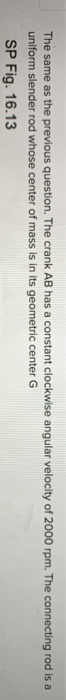The same as the previous question. The crank AB has a constant clockwise angular velocity of 2000 rpm. The connecting rod is a uniform slender rod whose center of mass is in its geometric center G SP Fig. 16.13 に8 in. 40° Check All That Apply ав [-10966, 227 cos 40 -10966.227 sin 40j - 0- -10966. 227 sin 40 + a)BD T, sinß + αΒί cosp -10966. 227 cos 4...

• #### state whether the graph crosses the x-axis or touches the x-axis and turns around at each zero

f(x)= -4(x+2)(x-5)3

• #### A woman with mass 50 kg is standing on the rim of a large disk that is rotating at 0.80 rev/s about an axis through its...

A woman with mass 50 kg is standing on the rim of a large disk that is rotating at 0.80 rev/s about an axis through its center. The disk has mass 270 kg and radius 4.0 m. Calculate the magnitude of the total angular momentum of the woman–disk system. (Assume that you can treat the woman as a point.) Express your answer to two significant figures and in...

• #### All of t rod of mass B. 2a _x10 kg and length C. 5meters, with two identical uniform hollow spheres, each of mass D. 232x 10 kg a E_ meters, attached at the ends of the rod, as shown below. Not...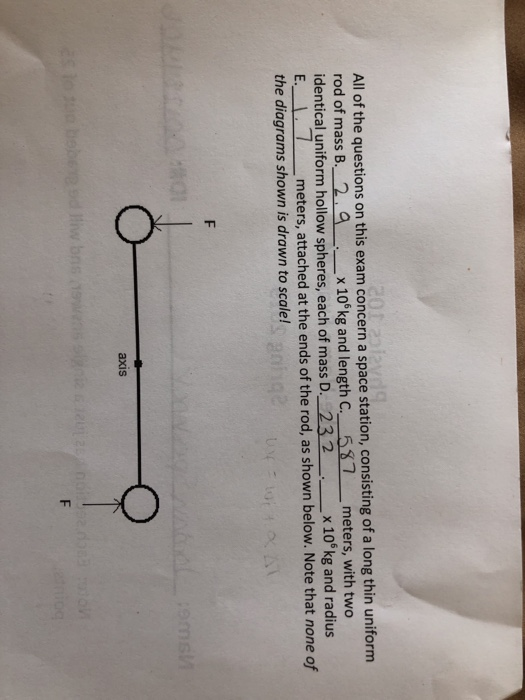All of t rod of mass B. 2a _x10 kg and length C. 5meters, with two identical uniform hollow spheres, each of mass D. 232x 10 kg a E_ meters, attached at the ends of the rod, as shown below. Note that none of the diagrams shown is drawn to scale! he questions on this exam concern a space station, consisting of a long thin uniform nd radius axis axis (e...

• #### please help 2. A metal rod with a length of 25.0 cm lies in the xz-plane and makes an angle of 15.00 with the positive x-axis and an angle of 75.0° with the positive z-axis. The rod is moving in t...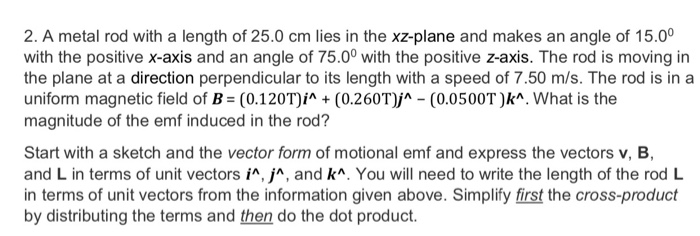please help 2. A metal rod with a length of 25.0 cm lies in the xz-plane and makes an angle of 15.00 with the positive x-axis and an angle of 75.0° with the positive z-axis. The rod is moving in the plane at a direction perpendicular to its length with a speed of 7.50 m/s. The rod is in a uniform magnetic field of B3(0.120T)/^ + (0.260T)/^-(0.0500T)k"...

• #### M,R a = 0 14. A body consisted of an uniform rod of mass m and length L, and a disk of mass M and radius R can rotate a...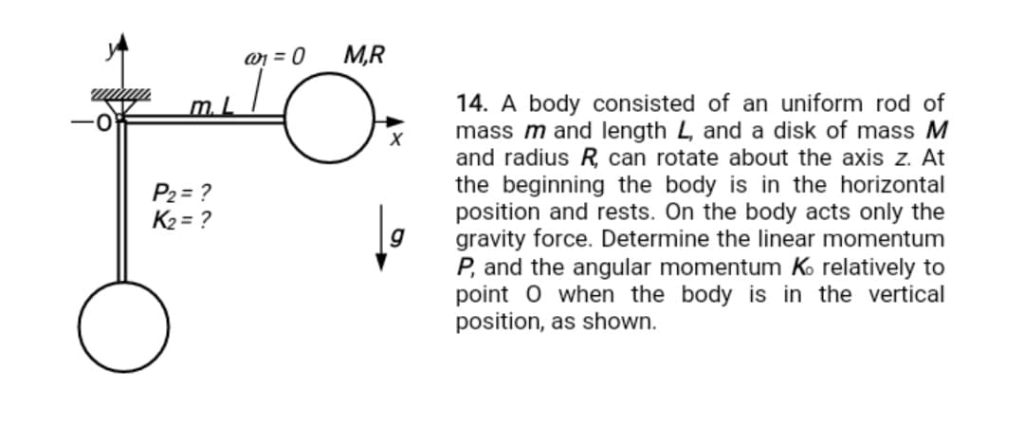M,R a = 0 14. A body consisted of an uniform rod of mass m and length L, and a disk of mass M and radius R can rotate about the axis z. At the beginning the body is in the horizontal position and rests. On the body acts only the gravity force. Determine the linear momentum P, and the angular momentum K relatively to point O when the body is in the vertical...

• #### A uniform disk of radius 0.42 m is mounted on a frictionless, horizontal axis

A uniform disk of radius 0.42 m is mounted on a frictionless, horizontal axis. A light cord wrapped around the disk supports a 1.2 kg object. When released from rest the object falls with a downward acceleration of 3.8 m/s2. The acceleration of gravity is 9.8 m/s2. What is the mass of the disk? Answer in kg.

• #### 44. The system shown in Fig. P7 consists of a slider block of mass m2 and a uniform slender rod of mass m3, length 13, and mass moment of inertia about its center of mass J The slider block is connec...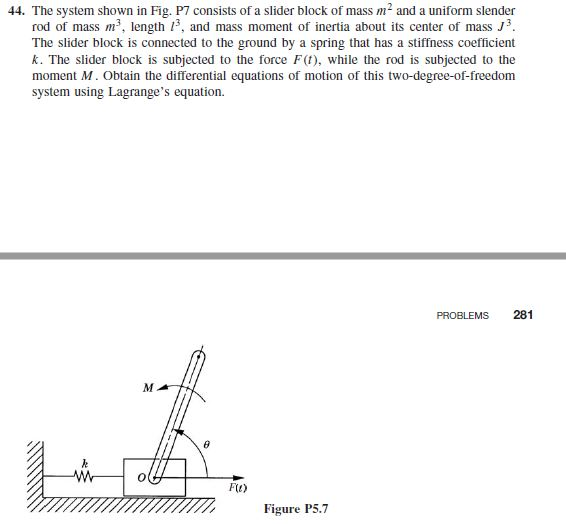44. The system shown in Fig. P7 consists of a slider block of mass m2 and a uniform slender rod of mass m3, length 13, and mass moment of inertia about its center of mass J The slider block is connected to the ground by a spring that has a stiffness coefficient k. The slider block is subjected to the force F(t), while the rod is subjected to the moment M. O...

• #### Question 2 2. In the figure below, the vane turns the water jet completely around. Find the maximum jet velocity Vo for a force Fo. (10 pts) 2. In the figure below, the vane turns the water jet...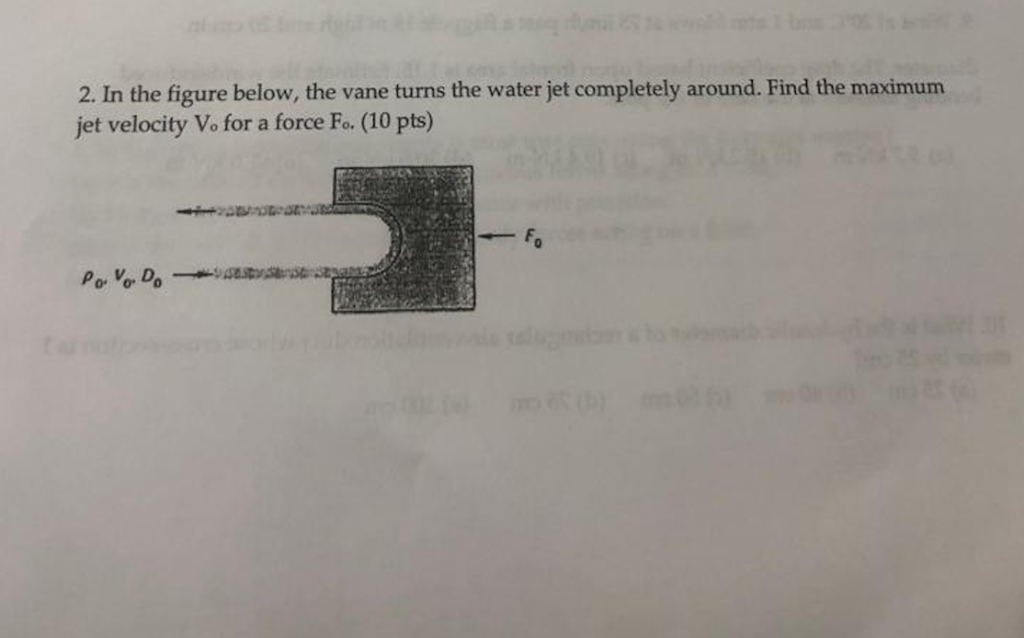Question 2 2. In the figure below, the vane turns the water jet completely around. Find the maximum jet velocity Vo for a force Fo. (10 pts) 2. In the figure below, the vane turns the water jet completely around. Find the maximum jet velocity Vo for a force Fo. (10 pts)

Need Online Homework Help?